## Kenya Certificate of Secondary Education (KCSE 2008) Chemistry Paper 1

1. A small crystal of potassium manganate (VII) was placed in a beaker containing water. The beaker was left standing for two days without shaking. State and explain the observations that were made. (2 marks)

2. When a hydrated sample of calcium sulphate CaSO4. XH2O was heated until all the water was lost, the following data was recorded:
Mass of crucible = 30.296 g
Mass of crucible + hydrated salt = 33.111 g
Mass of crucible + anhydrous salt = 32.781 g
Determine the empirical formula of the hydrated salt (Relative formula mass of CaSO4 = 136, H2O = 18). (3 marks)

3. Complete the following table by filling in the missing tests and observations. (3 marks)4. The structure of a detergent is1. What is the molecular formula of the detergent. (1 mark)
2. What type of detergent is represented by the formula? (1 mark)
3. When this type of detergent is used to wash linen in hard water, spots (marks) are left on the linen. Write the formula of the substance responsible for the spots (marks) (1 mark)

5. Phosphoric acid is manufactured from calcium phosphate according to the following equation.
Ca3(PO4)2(s) + 3H2SO4(l) ----> 2H2PO4(aq) + 3CaSO4(s)
Calculate the mass in (Kg) of phosphoric acid that would be obtained if 155 Kg of calcium phosphate reacted completely with the acid. (Ca = 40, P = 31, S = 32, O = 16, H =1)    (2 marks)

6. The structure below represents a sweet smelling compound.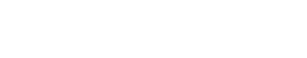Give the name of the two organic compounds that can be used to prepare this compound in the laboratory. (2 marks)

1. What are isotopes? (1 mark)
2. Determine the number of neutrons in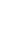(1 mark)

1. State the observation made at the end of the experiment when a mixture of iron powder and sulphur is heated in a test tube. (1 mark)
2. Write an equation for the reaction between the product in (a) above and dilute hydrochloric acid. (1 mark)
3. When a mixture of iron powder and sulphur is heated, it glows more brightly than that of iron fillings and sulphur. Explain the observation. (1 mark)

7. Zinc reacts with both concentrated and dilute sulphuric (VI) acid. Write equations for the two reactions. (2 marks)

8. When magnesium was burnt in air, a solid mixture was formed. On addition of water to the mixture a gas which turned moist litmus paper blue was evolved. Explain these observations. (2 marks)

9. The table below gives atomic numbers of elements represented by the letters A, B, C and D.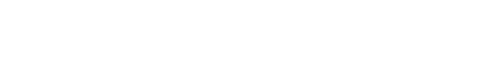Use the information to answer the questions that follow.
1. Name the type of bonding that exists in the compound formed when A and D react. (1mark)
2. Select the letter which represents the oxidizing agent. Give a reason for your answer. (2 marks)

10. In an experiment, a test-tube full of chlorine water was inverted in chlorine water as shown in the diagram below and the set up in sunlight for one day.After one day, a gas was found to have collected in the test-tube.
1. Identify the gas. (1 mark)
2. What will happen in the pH of the solution in the beaker after one day? Give an explanation. (2 marks)

11. In a laboratory experiment, hydrogen gas was passed over heated copper (II) oxide as shown in the diagram below.Describe a chemical test that can be used to identify the produce E. (2 marks)

12. Samples of urine from three participants F, G and H at an international sports meeting were spotted onto a chromatography paper alongside two from illegal drugs A1, Achromatogram was run using methanol. The figure below shows the chromatogram.1. Identify the athlete who had used an illegal drug. (1 mark)
2. Which drug is more soluble in methanol. (1 mark)

13. The table below gives  the solubilities of substances J, K and L at different temperatures.Select the substance which, when dissolved in water, heat is given out. Give a reason. (2 marks)

14. Starting with copper metal, describe how a sample of crystals of copper (II)  chloride may be prepared in the laboratory. (3 marks)

15. A compound whose general formula is M(OH)3 reacts as shown by the equations below.1. What is the name given to the compound which behaves like M(OH)3 in the two reactions. (1 mark)
2. Name two elements whose hydroxides behave like M. (2 marks)

16. The grid below is part of the periodic table. Use it to answer the questions that follow.
(The letters are not the actual symbols of the elements)1. Indicate on the grid the position of an element represented by letter whose atomic number is 14. (1 mark)
2. Select a letter which represents the monoatomic gas. (1 mark)
3. Write an equation for the reaction between Q and T. (1 mark)

17. The following are half-cell reactions and their reduction potentials.1. Write the cell representation for the electrochemical cell that would give the highest Eθ. (1 mark)
2. State and explain the observation made when copper rod is placed in a beaker containing silver nitrate solution. (2 marks)

1. State the Graham’s law of diffusion. (1 mark)
2. The molar masses of gases W and X are 16.0 and 44.0 respectively. If the rate of diffusion of W through a porous material is 12cm3 s -1. Calculate the rate of diffusion of X through the same material. (2 mark)

18. The diagram below represents an experiment that was set up to investigate movement of ions during electrolysis.When the circuit was completed, it was noticed that a blue colour spread towards the right.
1. Explain this observation. (2 marks)
2. Write the equation for the reaction that occurred in the anode. (1 mark)

19. The diagram below is a part of a set up used in the laboratory to prepare a gas.Complete the diagram to show how a sample of the gas can be collected. (3 marks)

20. In a closed system, aqueous iron (III) chloride reacts with hydrogen sulphide gas as shown in the equation below.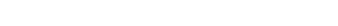State and explain the observation that would be made if dilute hydrochloric acid is added to the system equilibrium. (2 marks)

1. A radioactive substance emits three different particles.
Give the symbol for the particle with the highest mass. (1 mark)
1. Find the values of Z1 and Z2 in the nuclear equation below. (1 mark)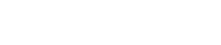2. What type of reaction is represented in b(i) above? (1 mark)

21. The graph below is a cooling curve of a substance from gaseous state to solid state.Give the name of the:
1. Process taking place between t0 and t1; (1 mark)
2. Energy change that occurs between t3 and t4. (1 mark)

22. When solid B1 was heated, a gas which formed a white precipitate  when passed through lime water was produced. The residue was then dissolved in dilute nitric (V) acid to form colourless solution B2. When the dilute hydrochloric acid was added to the solution B2 a white precipitate which dissolved on warming was formed.
1. Give the name of the:
1. Cation in the solid B1  (1 mark)
2. Anion in the solid B1  (1 mark)
2. Write an ionic equation for the reaction between the residue and dilute nitric (V) acid. (1 mark)

23.  In an experiment to determine the percentage of magnesium hydroxide in an anti-acid, a solution containing 0.50 g of the anti-acid was neutralized by 23.0 cm3 of 0.10 M hydrochloric acid.
(Relative formula mass of magnesium hydroxide = 58)
Calculate the:
1. Mass of magnesium hydroxide in the anti-acid; (2 marks)
2. Percentage of magnesium hydroxide in the anti-acid. (2 marks)

24. During the extraction of aluminium from its ores; the ore is first purified to obtain alumina.
The flow chart below shows the stages in the extraction of aluminium from alumina.1. Name:
1. Substance C1 (1 mark)
2. Process D1   (1 mark)
2. Give two reasons why aluminium is used extensively in the making of cooking pans. (1 mark)

25. A certain mass of metal E1 reacted with excess dilute hydrochloric acid at 25oC. The volume of the hydrogen gas liberated was measured after every 30 seconds. The results were presented as shown in the graph below.1. Name one piece of apparatus that may have been used to measure the volume of the gas liberated. (1 mark)
1. On the same axis, sketch the curve, that would be obtained if the experiment was repeated at 32oC.  (1 mark)
2. Explain the shape of your curve in b(i) above. (1 mark)

26. Crude oil contains sulphur. What would be the effect to the environment of using fuel containing sulphur? (1 mark)

27. Study the flow chart below and answer the questions that follow.1. Give the name for the process that takes place in step 1. (1 mark)
2. Give:
1. The name of substance G1. (1 mark)
2. One use of substance F1. (1 mark)